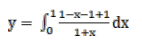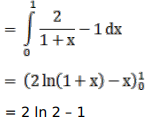# Mark against the correct answer in the following:

Question:

Mark $(\sqrt{)}$ against the correct answer in the following:

$\int_{0}^{1} \frac{(1-x)}{(1+x)} d x=?$

A. $\frac{1}{2} \log 2$

B. $(2 \log 2+1)$

C. $(2 \log 2-1)$

D. $\left(\frac{1}{2} \log 2-1\right)$

Solution: